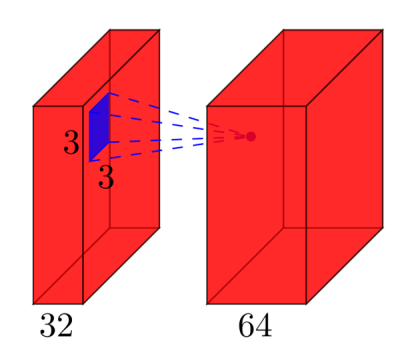# How To Calculate The Number Of Parameters For The CNN (Convolutional Neural Network)?

Let’s first look at how the number of learnable parameters is calculated for each individual type of layer you have, and then calculate the number of parameters in your example.

• Input layer: All the input layer does is read the input image, so there are no parameters you could learn here.
• Convolutional layers: Consider a convolutional layer which takes `l` feature maps at the input, and has `k` feature maps as output. The filter size is `n` x `m`. For example, this will look like this:Here, the input has `l=32` feature maps as input, `k=64` feature maps as output, and the filter size is `n=3` x `m=3`. It is important to understand, that we don’t simply have a 3×3 filter, but actually a 3x3x32 filter, as our input has 32 dimensions. And we learn 64 different 3x3x32 filters. Thus, the total number of weights is `n*m*k*l`. Then, there is also a bias term for each feature map, so we have a total number of parameters of `(n*m*l+1)*k`.

• Pooling layers: The pooling layers e.g. do the following: “replace a 2×2 neighborhood by its maximum value”. So there is no parameter you could learn in a pooling layer.
• Fully-connected layers: In a fully-connected layer, all input units have a separate weight to each output unit. For `n` inputs and `m` outputs, the number of weights is `n*m`. Additionally, you have a bias for each output node, so you are at `(n+1)*m` parameters.
• Output layer: The output layer is a normal fully-connected layer, so `(n+1)*m` parameters, where `n` is the number of inputs and `m` is the number of outputs.

The final difficulty is the first fully-connected layer: we do not know the dimensionality of the input to that layer, as it is a convolutional layer. To calculate it, we have to start with the size of the input image, and calculate the size of each convolutional layer. In your case, Lasagne already calculates this for you and reports the sizes – which makes it easy for us. If you have to calculate the size of each layer yourself, it’s a bit more complicated:

• In the simplest case (like your example), the size of the output of a convolutional layer is `input_size - (filter_size - 1)`, in your case: 28 – 4 = 24. This is due to the nature of the convolution: we use e.g. a 5×5 neighborhood to calculate a point – but the two outermost rows and columns don’t have a 5×5 neighborhood, so we can’t calculate any output for those points. This is why our output is 2*2=4 rows/columns smaller than the input.
• If one doesn’t want the output to be smaller than the input, one can zero-pad the image (with the `pad` parameter of the convolutional layer in Lasagne). E.g. if you add 2 rows/cols of zeros around the image, the output size will be (28+4)-4=28. So in case of padding, the output size is `input_size + 2*padding - (filter_size -1)`.
• If you explicitly want to downsample your image during the convolution, you can define a stride, e.g. `stride=2`, which means that you move the filter in steps of 2 pixels. Then, the expression becomes `((input_size + 2*padding - filter_size)/stride) +1`.

In your case, the full calculations are:

``  #  name                           size                 parameters---  --------  -------------------------    ------------------------  0  input                       1x28x28                           0  1  conv2d1   (28-(5-1))=24 -> 32x24x24    (5*5*1+1)*32   =     832  2  maxpool1                   32x12x12                           0  3  conv2d2   (12-(3-1))=10 -> 32x10x10    (3*3*32+1)*32  =   9'248  4  maxpool2                     32x5x5                           0  5  dense                           256    (32*5*5+1)*256 = 205'056  6  output                           10    (256+1)*10     =   2'570``

So in your network, you have a total of 832 + 9’248 + 205’056 + 2’570 = 217’706 learnable parameters, which is exactly what Lasagne reports.